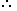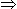# Easy Tricks to Solve Problems on Ages## Important Formulas on “Problems on Ages” :

1. If the current age is x, then n times the age is nx.

2. If the current age is x, then age n years later/hence = x + n.

3. If the current age is x, then age n years ago = x – n.

4. The ages in a ratio a : b will be ax and bx.

 5. If the current age is x, then 1 of the age is x . n n

## Problems on Ages -General Questions

1. Father is aged three times more than his son Ronit. After 8 years, he would be two and a half times of Ronit’s age. After further 8 years, how many times would he be of Ronit’s age?
A. 2 times
B.
 2 1 times 2
C.
 2 3 times 4
D. 3 times

Explanation:

Let Ronit’s present age be x years. Then, father’s present age =(x + 3x) years = 4x years.(4x + 8) = 5 (x + 8) 28x + 16 = 5x + 403x = 24x = 8.

 Hence, required ratio = (4x + 16) = 48 = 2. (x + 16) 24
2. The sum of ages of 5 children born at the intervals of 3 years each is 50 years. What is the age of the youngest child?
 A. 4 years B. 8 years C. 10 years D. None of these

Explanation:

Let the ages of children be x, (x + 3), (x + 6), (x + 9) and (x + 12) years.

Then, x + (x + 3) + (x + 6) + (x + 9) + (x + 12) = 505x = 20x = 4.Age of the youngest child = x = 4 years.

## Solve the following Aptitude Problems on Ages

Correct! Wrong!

Correct! Wrong!

Correct! Wrong!

Correct! Wrong!

#### Six years ago, the ratio of the ages of Kunal and Sagar was 6 : 5. Four years hence, the ratio of their ages will be 11 : 10. What is Sagar's age at present?

Correct! Wrong!

Quiz on Problems of Ages
GREAT JOB!!!